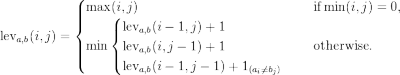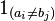Jans Blog

## Programming

15.09.2020

### Motivation

My two Android apps Metal Only and MensaUPB require some kind of online service to get their data from and - since I cannot force users to update apps easily - I wrote my own API as a wrapper to the upstream APIs provided by https://www.metal-only.de/ and https://studierendenwerk-pb.de/. That way, whenever one of these pages changes their API, I can just adapt the wrapper and keep my own API backwards-compatible so the apps themselves don’t require any updates.

The current version of the API was developed with Play! Scala. However, due to my interest in the Rust programming language I considered a rewrite. An additional advantage of switching to Rust would be the potential of switching to running the app on-demand instead of keeping it always running: a restart of the current Play! Scala API takes around 3~5 seconds.

### Plan

The rewrite will be split in multiple phases due to limitted time.

The Experimental Phase will just consist of developing multiple proof of concepts to see how viable using Rust for the rewrite is. This entails querying upstream APIs and providing a REST API which can be used by my apps.

In the Cleanup Phase unused endpoints of the current API will be removed which is useful even without a rewrite. The fastest way to do this cleanup was quite simple:

• Add a logging plugin to the heroku app and enable email alerts whenever the app serve a “HTTP 404 Not Found” error
• Remove all duplicate and deprecated routes which are unused by current versions of my apps
• Re-add all routes that turn out to be still required, i.e. valid requests that produced a HTTP 404

The Rewrite Phase is most likely over-engineered and consists of two parts: an integration test and the actual apps. The integration test is used to ensure that the rewrite works as the currently existing app which is currently tested in production. The basic idea here is to replace the upstream APIs with simulated data (“mocks”). The first step here was to make the upstream API configurable.

Since there was never a requirement to replace the upstream API’s URL, there was no option to do so. The option was added as an environment variable and - if the environment variable is not set - defaults to the hard-coded URL (pseudo-code):

``````url = get_environment_variable("UPSTREAM_URL")
if is_not_set(url) {
url = DEFAULT_UPSTREAM_URL
}
``````

Additionally, I wanted to use this rewrite to further my skills with Docker and docker-compose: the rewritten API, the current API, the integration test, and the mocked upstream APIs should all be started with a single command, i.e. `docker-compose up app-api-rewrite`.

### Current State

The rewrite started in March 2023 and was paused for a few months. While experimenting and cleaning up were done quite fast, the rewrite itself is still in progress. One of the major factors is the deliberate over-engineering.

30.12.2014

### Cleaning some data with Clojure 3

The first post in this series was about the original problem: there is a data-set of strings with potential duplicates and many typos. The second was about computing the similarity of two strings. In this post we will focus on the question

Can we group multiple strings of a set by similarity?

Matching the names: The first step is to match the names. I hope, that the following code is documented good enough.

```(defn- singleMap
"Produces a function that takes one argument str2 and computes
[str1 str2 (f str1 str2)]."
[f str1]
(fn [str2]
[str1 str2 (f str1 str2)]))

(defn- split?
"Produces a split predicate based on a."
[str]
(fn [x]
(pos? (compare str x))))

(defn- getAfterStr
"Returns the elements after str of the supplied sequence"
[str strings]
(get (split-with (split? str) strings) 1))

(defn- singleMapWAll
"Produces a producer function that maps the matching function
with a to all elements after a."
[f strings]
(fn
[str1]
(map (singleMap f str1) getAfterStr)))

(defn match
"Uses the given similarity function f and matches all
strings with each other string once"
[f strings]
; Applys the function produced by singleMapWAll to strings
; and concatenates the result
(mapcat (singleMapWAll f strings) strings))
```

The following table provides some more information. a, b, c, d, and e are the strings to be matched. “f str1” denotes that a function “f str1” is mapped onto the corresponding string in the last row (which will be used as str2 in the matching).

 (f d) (f c f c) (f b f b f b) (f a f a f a f a) a b c d e

Whereas map would produce a mapping as above, mapcat applys the mapping and concatenates the results as displayed below.

 (f a f a f a f a f b f b f b f c f c f d) a b c d e c d e d e e

After all matching fs are applied, we get our result tuples in one list:

 ([a b (f a b)] [a c (f a c)] … [d e (f d e)])

Filtering the results: This filtering step is just an intermediate step. We will use the results find good “grouping” values. Remember that a result vector has the form `[str1 str2 (similarity str1 str2)`.

```(defn- minSimFilterBuilder
[min-sim]
(fn [x]
(>= (get x 2) min-sim)))

(defn- unequalName?
[x]
(not (= (get x 0) (get x 1))))

(defn cleanMatches
[matches min-sim]
(filter (minSimFilterBuilder min-sim)
(filter unequalName? matches)))
```

`minSimFilterBuilder` is used to construct filter functions based on a minimum similarity value. The function `unequalName?` simply checks, if the names of the result tuple are different. The result of `cleanMatches` will then contain only matches with distinct strings that are “similar enough” for us.

27.12.2014

### Cleaning some data with Clojure 2

Cleaning some data with Clojure 1” provides some context on this project. This post here focusses on the question

“Can we tell, if two strings are similar?”.

Levenshtein Distance: My first idea was to use the Levenshtein Distance. This metric counts the minimum number of operations needed to transform one string into the other when allowing insertion, deletion or substition of characters. Its definition is as follows:<figcaption class="wp-caption-text">Definition of Levenshtein Distance</figcaption></figure>denotes the indicator function, which is equal to 0 when the i-th character of a is equal to the j-th character of b. Otherwise it’s 1.

Transforming this into clojure-code is fairly simple, given the definitions. First, the indicator-function:

```(defn indicator
"0, if the i-th character of a and the j-th character of b
are the same. 1 otherwise."
[a b i j]
(if (= (get a (dec i)) (get b (dec j)))
0
1))
```

Next, a helper function which will be called recursively:

```(defn lev
"Helper function to compute the levenshtein distance"
[a b i j]
(if (zero? (min i j))
(max i j)
(min (inc (lev a b (dec i) j))
(inc (lev a b i (dec j)))
(+ (lev a b (dec i) (dec j)) (indicator a b i j)))))```

And finally the “public api”:

```(defn levenshtein
"Computes the Levenshtein distance between two strings a and b"
[a b]
(lev a b (count a) (count b)))
```

Unfortunately, this solution is not very performant . Also it blows the stack somewhere above 50 characters (tested for 140 characters). Nonetheless, this is enough for my use-case 😉

```=> (def alphabet "alphabetdefghijklmnopqrstuvwxyz")
#'/alphabet
=> (time (levenshtein (subs alphabet 0 10) (subs alphabet 0 10)))
"Elapsed time: 3855.856306 msecs"
0
=> (time (levenshtein (subs alphabet 0 11) (subs alphabet 0 11)))
"Elapsed time: 21493.424412 msecs"
0
```

We have this performance issue because most values are computed multiple times; some are computed extremely often. The solution in this case is a well-placed `memoize` to reuse the computed values: `(def lev (memoize lev))`. All in all we get a huge performance boost :

```=> (time (levenshtein (subs alphabet 0 11) (subs alphabet 0 11)))
"Elapsed time: 1.230304 msecs"
0
=> (time (levenshtein (subs alphabet 0 15) (subs alphabet 0 15)))
"Elapsed time: 1.280788 msecs"
0
=> (time (levenshtein alphabet alphabet))
"Elapsed time: 3.099704 msecs"
0
=> (time (levenshtein rnd_50_1 rnd_50_2))
"Elapsed time: 11.464978 msecs"
38
=> (time (levenshtein rnd_140_1 rnd_140_2))
StackOverflowError clojure.lang.AFn.applyToHelper (AFn.java:148)
```

Note, that “rnd_X_Y” are distinct pseudo-random strings that were “generated” by hitting the keyboard X times.

Extending this metric: This metric does not take into account that \”o is equivalent to ö. We can fix this by replacing these special values with more common ones before computing the distances:

```(defn cleanString
"A hacky cleaning function. Replaces unusual characters by more common ones."
[s]
(switch "\"A" "Ä"
(switch "\"O" "Ö"
(switch "\"U" "Ü"
(switch "\"a" "ä"
(switch "\"o" "ö"
(switch "\"u" "ü"
(switch "\\ss{}" "ß" s))))))))))))
```

And for a vector etc. of strings:

```(defn clean
"Cleans a vector of strings"
[strings]
(map cleanString strings))
```

From distance to similarity: Our next step is to convert distances between two strings into a “similarity value”. The Levenshtein distance of two strings is `(<= 0 (levenshtein a b) (max (count a) (count b)))`. Therefore we can convert a Levenshtein distance to a similarity metric as follows:

```(defn levensthein-similarity
"Computes a similarity measure based on Levenshtein distance. 0 ~ a and b completely different, 1 ~ a and b equal"
[a b]
(- 1 (/ (levensthein a b)
(max (count a) (count b)))))
```

TL;DR: Use an appropriate String Metric and convert it to a similarity metric. I tried out Levenshtein Distance which does not takes into account that \”o and ö are equivalent.

 “We should forget about small efficiencies, say about 97% of the time: premature optimization is the root of all evil.” (Knuth, Donald. Structured Programming with go to Statements, ACM Journal Computing Surveys, Vol 6, No. 4, Dec. 1974. p.268.); from clean-code-developer.de

 These numbers mean next to nothing: we don’t know yet if these improvements hold true for random strings. This will probably be on a later post.

Edit: Better code listings.

25.12.2014

### Cleaning some data with Clojure 1

Just playing around with some distance measures for strings and some test data. Post ganz lesen